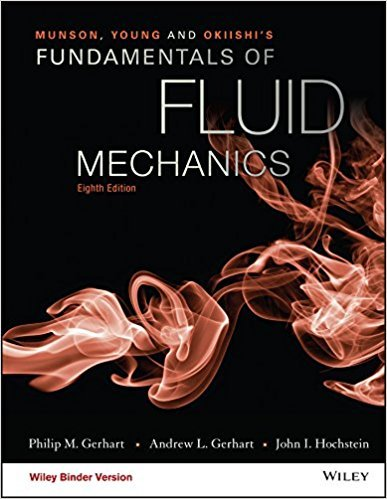×
×

# An expression for the value of cp for carbon dioxide as afunction of temperature iscp =ISBN: 9781119080701 456

## Solution for problem 11.8 Chapter 11.1

Fundamentals of Fluid Mechanics | 8th Edition

• Textbook Solutions
• 2901 Step-by-step solutions solved by professors and subject experts
• Get 24/7 help from StudySoup virtual teaching assistantsFundamentals of Fluid Mechanics | 8th Edition

4 5 1 263 Reviews
24
1
Problem 11.8

An expression for the value of cp for carbon dioxide as afunction of temperature iscp = 286 1.15 105T+2.49 106T2where cp is in (ft lb)/(lbm R) and T is in R. Compare thechange in enthalpy of carbon dioxide using the constant value of cp(see Table 1.7) with the change in enthalpy of carbon dioxide usingthe expression above, for T2 T1 equal to (a) 10 R, (b) 1000 R,(c) 3000 R. Set T1 = 540 R.

Step-by-Step Solution:
Step 1 of 3

Behavior = “any internally coordinated, externally visible pattern of activity that responds to changing external or internal conditions” Tinbergen’s Four Questions  Two levels of analysis: o Proximate cause: How behavior occurs o Ultimate: why behavior occurs  Four types: o Proximate (more short...

Step 2 of 3

Step 3 of 3

##### ISBN: 9781119080701

Unlock Textbook Solution